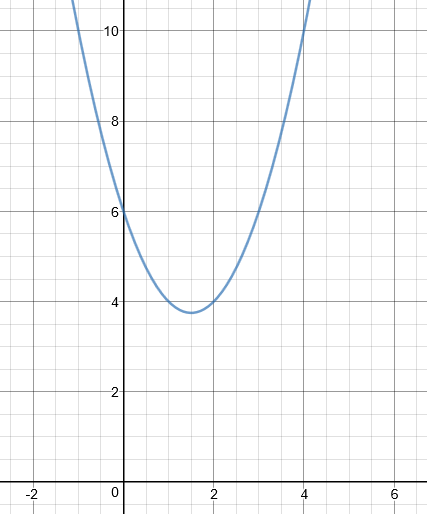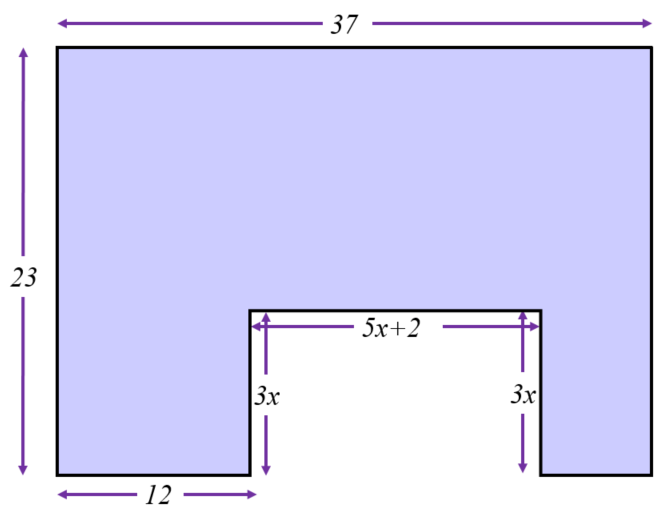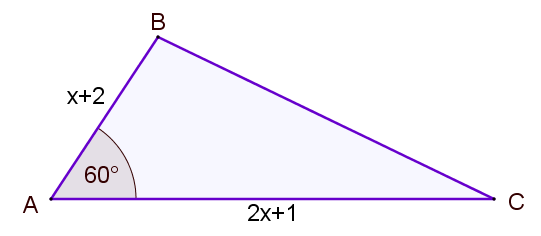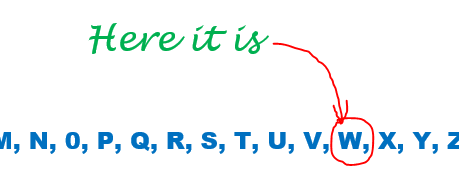# Exam-Style Questions.

## Problems adapted from questions set for previous Mathematics exams.

### 1.

GCSE Higher

The diagram shows part of the graph $$y=x^2-3x+6$$.(a) By drawing a suitable straight line, use your graph to find estimates for the solutions of $$x^2 - 4x + 2 = 0$$ to one decimal place.

(b) A is the point (2,4). Calculate an estimate for the gradient of the graph at the point A.

### 2.

IGCSE Extended

(a) Show that the equation $$\frac{3}{x+1}+\frac{3x-9}{2}=1$$ can be simplified to $$3x^2-8x-5=0$$.

(b) Solve the equation $$3x^2-8x-5=0$$ showing all of your working and giving answers to three significant figures.

(c) The total surface area of a cone with radius $$x$$ and slant height $$8x$$ is equal to the area of a circle with radius r. Show that $$r = 3x$$.

[The curved surface area, $$A$$, of a cone with radius $$r$$ and slant height $$l$$ is $$A=\pi rl$$.]

### 3.

GCSE Higher

In the diagram below, which is not drawn to scale, all dimensions are in centimetres and all angles are multiples of 90o. If the shaded area is 698cm2, work out the value of $$x$$.### 4.

GCSE HigherThe area of triangle ABC (not drawn to scale) is

$$\frac{35 \sqrt{3}}{4} m^2$$

If AB = $$x+2$$ metres and AC = $$2x+1$$ metres, find the value of $$x$$ to three significant figures.

### 5.

GCSE Higher

If $$y = 5x^4 + 3x^2$$ and $$x=\sqrt{w+2}$$, find $$w$$ when $$y = 12$$ showing each step of your working.The exam-style questions appearing on this site are based on those set in previous examinations (or sample assessment papers for future examinations) by the major examination boards. The wording, diagrams and figures used in these questions have been changed from the originals so that students can have fresh, relevant problem solving practice even if they have previously worked through the related exam paper.

The solutions to the questions on this website are only available to those who have a Transum Subscription.

Exam-Style Questions Main Page

Search for exam-style questions containing a particular word or phrase:

To search the entire Transum website use the search box in the grey area below.